Rational exponentsPage 1

WATCH ALL SLIDES

Slide 1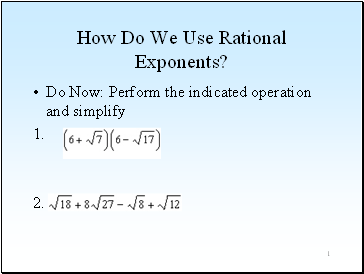How Do We Use Rational Exponents?

Do Now: Perform the indicated operation and simplify

Slide 2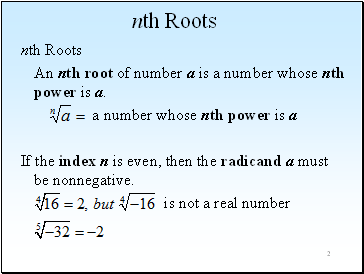2

nth Roots

nth Roots

An nth root of number a is a number whose nth power is a.

a number whose nth power is a

If the index n is even, then the radicand a must be nonnegative.

is not a real number

Slide 3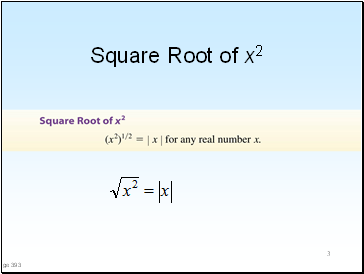3

Square Root of x2

Page 393

Slide 4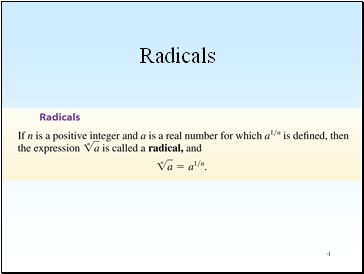4

Slide 55

Rational Exponents

Slide 6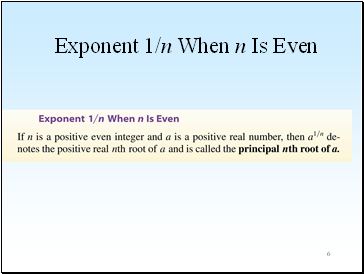6

Exponent 1/n When n Is Even

Slide 7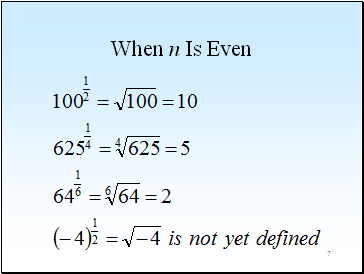7

When n Is Even

Slide 8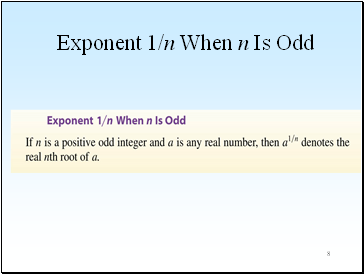8

Exponent 1/n When n Is Odd

Slide 9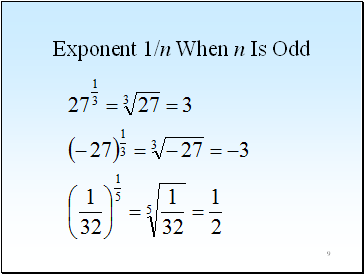9

Exponent 1/n When n Is Odd

Slide 10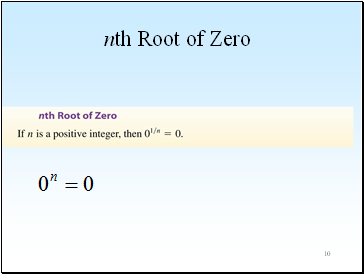10

nth Root of Zero

Slide 11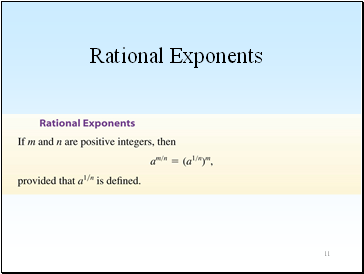11

Rational Exponents

Slide 12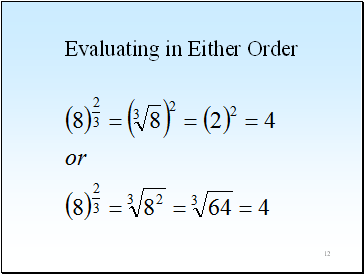12

Evaluating in Either Order

Slide 13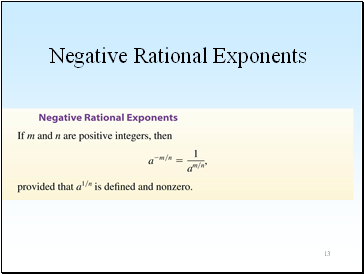13

Negative Rational Exponents

Slide 14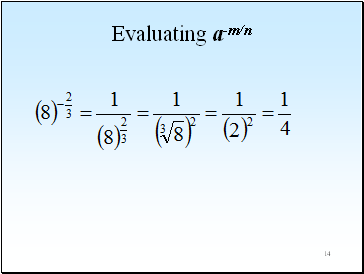14

Evaluating a-m/n

Slide 15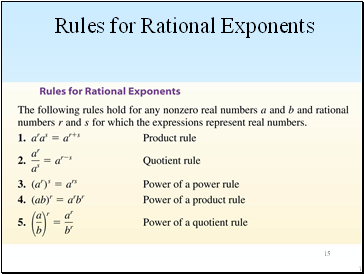15

Rules for Rational Exponents

7-6

Slide 16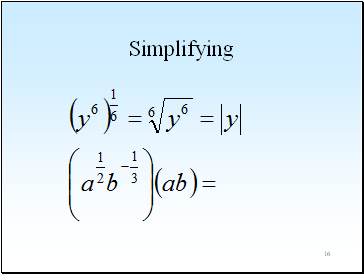16

Simplifying

Slide 17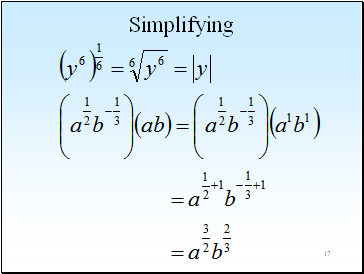17

Simplifying

Slide 18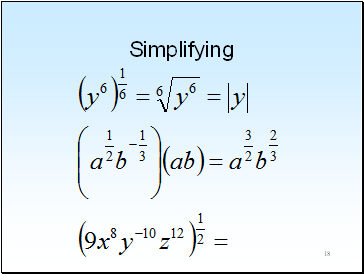18

Simplifying

Slide 19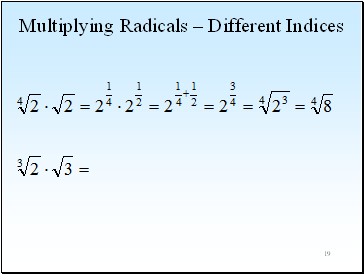19

Multiplying Radicals – Different Indices

Slide 20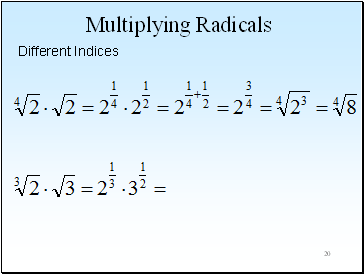20